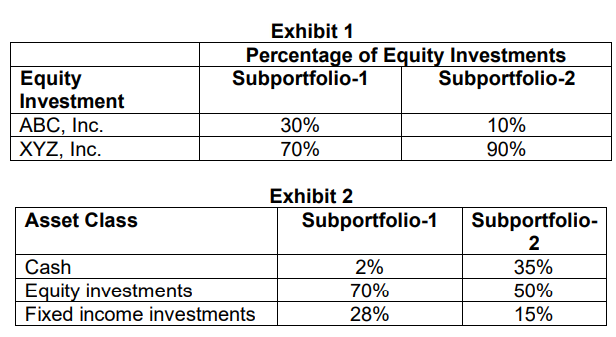# Equity allocation and HHI

Shannon Kay is an analyst with a leading pension manager. Kay is evaluating two subportfolios within the overall pension portfolio, both of which have their equity investment are evaluated against their style benchmarks. Subportfolio-1 is constructed using a fundamental approach focused on value stocks, while Subportfolio-2 is constructed using a quantitative approach focused on growth stocks. The equity portion of each subportfolio consists of just two stocks (ABC and XYZ) as shown in Exhibit 1. Each subportfolio invests in cash, equity and fixed income securities as shown in Exhibit 2.C. Discuss one negative component of the current asset allocation of Subportfolio- 2 that may impact its returns relative to its index benchmark.
D. Calculate the Herfindahl–Hirschman Index (HHI) for Subportfolio-1 and Subportfolio-2, Determine which of the subportfolios is more diversified based solely on the HHI calculations.

On C: besides cash drag, I also mentioned a concentrated stock position in XYZ, which could lead to a suboptimal risk-adjusted return. Is that wrong as a negative aspect to be mentioned here? I am asking this because they only included cash drag in the solution.

On D:

The effective number of stocks is a measure of diversification that is calculated by determining the inverse of HHI. Hence, HHI is 1/0.2842 = 3.52 and 1/0.205 = 4.88 for
Subportfolio-1 and Subportfolio-2, respectively. Therefore, based solely on this measure, Subportfolio-2 is more diversified.
So, the higher the HHI, the higher the concentration, meaning, strictly based on HHI, subportfolio 2 is more diversified, because it has a lower HHI of 0.205, correct? Do you even need to calculate the effective number of stocks here? Thanks!